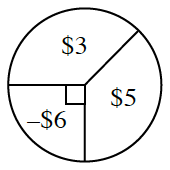### Home > CCG > Chapter 5 > Lesson 5.2.2 > Problem5-69

5-69.

The probability of winning $\3$ on the spinner at right is equal to the chance of winning $\5$. Find the expected value for one spin. Is this game fair?

Refer to the Math Notes box in this lesson.

Part of the calculation for the expected value is $3\left(\frac{135}{360}\right)$.

Is the game fair?
That means the amount you win is equal to the amount you lose, or the expected value is $0$.